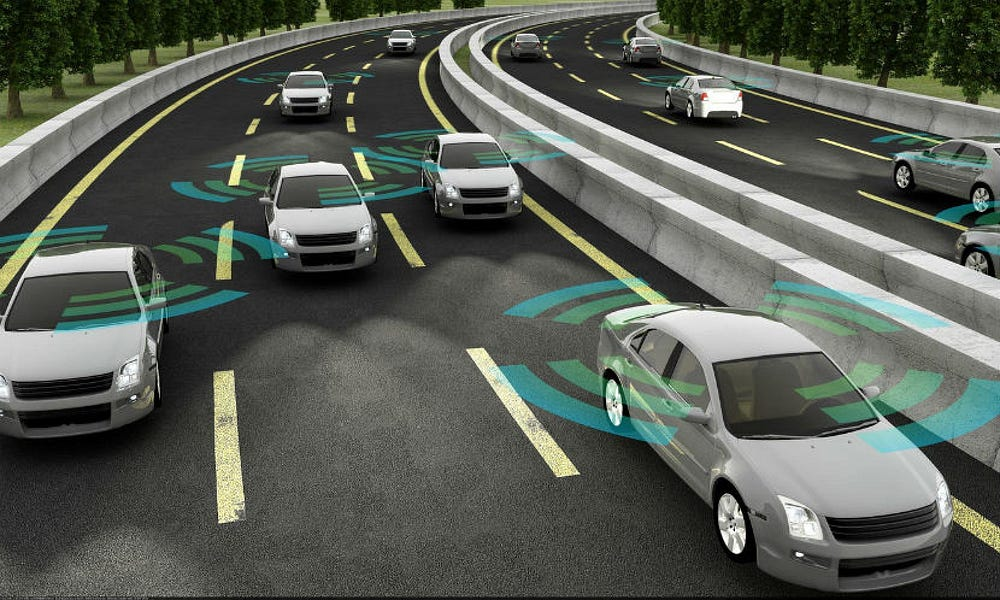# Autonomous Driving —  Finding Lane Lines on the Road

Original article was published on Deep Learning on MediumGiven that we have our expensive camera mounted on a fixed position on the car, we can expect the lane lines to always appear in the same general region. Let’s give this region a fancy name (The Region of Interest).

This region is somewhat triangular or polygonal in front of the car. We then define a mask to extract this region. Now think about that, we’ve made the problem even simpler. We only have a small region to consider.

We are now ready to detect lanes in our canny edge image. But, hold your brakes for a moment.

# Hough Transform

How do we know that several dots in our canny image form a line? We have to convert our detect edges (dots) in our canny image from linear space (Cartesian space of x, and y) to parametric space. Let’s honour the inventor of this method by calling it Hough Transform. Hough transform, transforms a point (x, y) on a line defined by the linear equation

y = mx + b

to a point in Hough space defined by the parameters m vs b. A line in Hough space is defined by the equation.

b = y — mx.

A line in an image space corresponds to a point (m, b) in a Hough space. Consequently, a line in a Hough space corresponds to a point (x, y) in image space.

Parallel lines in our image space will have the same slope (m) in Hough space but will intercept at different values (b).

Here is the beauty, given points in image space, we get lines in Hough space. And if these lines intersect in Hough space, it means the points in the image space forms a line.

## How to Handle Vertical Lines

Just a little problem. Vertical lines in an image space have an infinite slope (m) which will be difficult to represent in a Hough space. Hence, in the Hough space instead of plotting m vs b, to solve this problem, we we plot θ vs ρ (Polar coordinates). Theta is the angle inclination of the line from horizontal and rho the distance from the origin.

The advantage of the Hough transform is that it allows finding disconnected points that form a single continuous lane. How coupled should this point be for us to consider that that make up a line? You decide, using the threshold parameter in the algorithm.

What if the algorithm return a single dot as forming a line, it that acceptable? Well, you will have to decide the minimum number of pixels that could form a line using another parameter min_line_length.

Also, if two lines are disconnected by just a few pixels, will we not want the algorithms to join these lines as on one. Yes, we can do that using another parameter max_line_gap to specify the maximum gap between line segments to be considered one.

These parameters are what we will need when using the Hough algorithm.

Luckily for us, all these algorithms have been implemented in the Open Source Computer Vision library (OpenCV). We just need to plug in our parameters.

These are just what we need to know to successfully implement a pipeline to detect lane lines. I have pieced all of this together in the project here. Here is the result of our original image passed through our pipeline.